medium.com Go back Open original

# Einstein’s 1905 paper on the Photoelectric Effect - Cantor’s Paradise

By Jørgen Veisdal

Although by 1905 the state of experimental techniques in particle physics were still rudimentary, what are still considered important discoveries had already been made (Pais, 1979). Among these were the photoelectric effect, discovered by Heinrich Hertz (1857–94) in 1887 by accident when he was studying the electromagnetic wave nature of light:

`"At one point [Hertz] was studying spark discharges generated by potential differences between two metal surfaces. A primary spark coming from one surface generated a secondary spark on the other. Since the latter was harder to see, Hertz built an enclosure around it to eliminate stray light. He was struck by the fact that this caused a shortening of the secondary spark. He found next that this effect was due to that part of the enclosure which was interposed between the two sparks. It was not an electrostatic effect since it made no qualitative difference whether the interposed surface was a conductor or an insulator."Excerpt, "Einstein and the quantum Theory" by Abraham Pais (1979)`

Hertz began to suspect that the secondary spark might be due to the light given off by the primary spark, and began conducting tests to confirm his suspicion. He found and confirmed that light can indeed produce sparks, and reported his findings in the paper Ueber einen Einfluss des ultravioletten Lichtes auf die electrische Entladung in Annalen der Physik, but did not pursue the investigation further.

## Einstein’s Paper

Einstein opened his own investigation into Hertz’ observed effect by pointing out that James Clark Maxwell’s classic theory of electromagnetic radiation differs profoundly from the theoretical models of gases and other matter. Namely, the former theory specifies that electromagnetic regions of space are described by continuous functions which by definition cannot be described by any finite number of variables. Thus, according to Maxwell’s theory, the energy of purely electromagnetic phenomena (such as light) should be represented as a continuous phenomena. That is, as opposed to the energy of other phenomena such as matter which should be represented by discrete sums of atoms and electrons.

Acknowledging the usefulness of Maxwell’s wave theory of light for representing purely optical phenomena, Einstein argues that “we should consider that optical experiments observe only time-averaged values” and so despite the perfect agreement of Maxwell’s theory with observations from experiments, “the use of continuous spatial functions to describe light may lead to contradictions with experiments, especially when applied to the generation and transformation of light”. In particular, Einstein highlights observations of black body radiation, photoluminescence and the production of cathode rays by ultraviolet light as indicative of this fact.

In other words, Einstein opens his paper with the claim that although treated as a continuous phenomena in Maxwell’s theory of electromagnetism, various experimental observations indicate that “the energy of light” appears to not be continuous, but rather “distributed discontinuously in space”.

## A Certain Problem Concerning the Theory of “Black Body Radiation”

To substantiate his claim, Einstein next narrowed in especially on the concept of black body radiation, which had in essence been understood by 1900, the year Max Planck discovered the black body radiation law, “Planck’s Law” (Pais, 1979):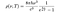Equation 1. Planck’s Law describing the energy density of electronmagnetic radiation emitted by a black body in thermal equilibrium in a volume at a given temperature T when there is no net flow of matter or energy between the body and its environment.

Black body radiation is the thermal electromagnetic radiation within or surrounding a body in thermodynamic equilibrium, emitted by a black body (a theoretical, opaque, non-reflective body). Think of a black body as one that absorbs all the electromagnetic radiation (“light”) that strikes it, and emits radiation at the same rate, in the form of heat in order to remain in thermal equilibrium. Radiation at room temperature is at infrared wavelengths (and so invisible to the naked eye), but as the body gets hotter, radiation begins to become visible as red, orange, yellow, white and blue with increasing temperature. Imagine the color range of molten magma at various temperatures as an example of this phenomenon.

Einstein’s investigation sets out with a discussion of “a difficulty” in the theory of black body radiation by noting that Planck’s law (eq. 1) appears to agree with experiments, but is inconsistent with other established concepts in classical physics. As Pais (1979) notes, Einstein’s simple argument is based on two known concepts from classical theory:

• One of Planck’s equations (1897), in which the quantity U represents the equilibrium energy level of a one-dimensional harmonic oscillator at a frequency v is the frequency and temperature T: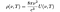Equation 2. Planck’s equation, giving energy density as a function of frequency v and temperature T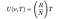Equation 3. Equilibrium energy level of a one-dimensional harmonic oscillator

Very simply, from these two equations, substituting for U(v,T) Einstein obtained the following equation which later became known as the Rayleigh-Jeans law: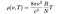Equation 4. The Rayleigh-Jeans law, giving energy density as a function of frequency v and temperature T

Einstein’s claim was simple: although Planck’s Law (eq. 1) agreed with experiment, Einstein could not get it to agree with extant classical theory as of 1905, despite his most arduous efforts:

“All my attempts … to adapt the theoretical foundations of physics to this [new type of] knowledge failed completely. It was as if though the ground had been pulled out from under one, with no firm foundation to be seen anywhere.” — Einstein (1949a)

## Thought experiment

Einstein illustrates the discrepency by imagining a cavity with perfectly reflecting walls, filled with freely moving electrons and gas molecules. In addition, the cavity is filled with a number of electrons bound to spatially seperated points by forces that increase linearly with seperation, which he calls “resonators” since they absorb and emit electromagnetic waves of a particular period. He points out that according to the theory of the generation of light, the radiation in the cavity must be identical to black body radiation, “at least if one assumes that resonators exist for every frequency under consideration”.

First, he considers the requirement of thermal equilibrium for black bodies, namely that the temperature of the cavity is spatially uniform and the temperature is constant. According to the kinetic theory of gases, dynamic equilibrium requires that the average kinetic energy of a resonator (electrons bound to spatially seperated points) equals the average kinetic energy of a freely moving gas molecule. Decomposing the motion of such a resonator into three mutually perpendicular oscillations (movement in three spatial dimensions), Einstein finds that the average energy of such a linear oscillation for a resonator electron must be given by:

where R is the gas constant, N is Avogadro’s constant and T is the absolute temperature of the electron. Because of the time averages of the kinetic and potential energy, he argues that the energy Ē is 2/3 as large as the kinetic energy of a single free gas molecule. “Even if something such as radiative processes causes the time-averaged energy of a resonator to deviate from the value Ē, collisions with free electrons and gas molecules will return its average energy to Ē by absorbing or releasing energy”. Hence, dynamic equilibrium in the system can only exist when every resonator has an average energy Ē.

Having established the energy level of a resonator electron according to statistical mechanics, Einstein next moves on to a similar consideration of changes in the energy of the resonator electron when interacting with the ambient radiation within the cavity. He applies Planck’s equation for the average energy of a resonator of frequency v: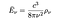Equation 6. Average energy of an electron resonating at frequency v

where rho ρ denotes the energy density of the cavity’s radiation. Einstein points out that if the net energy of the electron resonating at frequeny v is not to continually increase or decrease (violating the condition for dynamic equilibrium), the following equality must hold: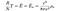Equation 7. Condition for dynamic equilibrium of a resonating electron, given by its temperature T, the gas constant R, Avagadro’s constant N, the frequency of its oscillations v and the energy density ρ of the radiation

Which, rewritten may be stated asEquation 4. The Rayleigh-Jeans law, giving energy density as a function of frequency v and temperature T

Equation 4 is a condition for dynamic equilibrium which Einstein funnily states “not only lacks agreement with experiment, it also eliminates any possibility of equlibrium between matter and aether.” The wider the range of frequencies chosen, the bigger the radiation energy in the space becomes and in the limit one obtains: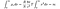Equation 8. Energy density in the limit, as the frequency v of the resonance of an electron approaches infinity

Disastrous. Einstein had shown that Planck’s equation of 1897 giving the condition for dynamic equilibrium of a black body was inconsistent with the known and experimentally verified theory giving the correct energy of harmonic oscillations of electrons.

“If Planck had drawn this conclusion, he would probably not have made his great discovery” (Einstein, 1949a)

## Molecular Theoretical investigation of the Volume Dependence of the Entropy of Gases and Dilute Solutions

Einstein’s discussion next set out to revise the theory of black body radiation im Anschluss an die Erfahrung (“in connection with experiment”), based on what experiments thus far had indicated. The experimental information Einstein made use of was the validity of something called Wien’s law (1896) in replace of Planck’s equation (eq. 2). Wien’s equation may be written as:

Einstein chose Wien’s law as the outset of his investigation because “the observations of black body radiation so far indicate that the law that Mr. Wien originally devised […] for large v/T [short wavelength/high frequency spectrum of thermal emissions] experiments completely confirm the law”. As Pais (1979) writes:

`"Einstein extracted the light-quantum postulate from this law by drawing an analogy between radiation in the Wien regime and a gas of (classical) noninteracting point particles, often called an ideal Boltzmann gas. Specifically he made use of the volume dependence of the entropy for such a gas."Excerpt, "Einstein and the quantum theory" by Abraham Pais (1979)`

Einstein derived the latter relation (between entropy and volume) in the following way, from the second law of thermodynamics and the ideal gas law starting with Boltzmann’s entropy formula (Pais, 1979):

According to the equation, a reversible change from the state a to another state b satisfies the following relation where R is the gas constant, N is Avagadro’s number and W is the number of microstates corresponding to the gas’ macrostate: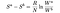Equation 11. The change between states a and b according to Boltzmann’s relation (eq. 10)

Einstein next considers a system consisting of subsystems 1, 2, …, which do not interact and therefor are statistically independent. Then W = W₁×W₂×…, and so the change between states a and b in the system is given by: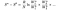Equation 12. The change between states a and b according to Boltzmann’s relation (eq. 10) for a system consisting of subsystems 1, 2, …

For the case of an ideal Boltzmann gas (theoretical gas composed of many randomly moving particles whose only interactions are perfectly elastic collisions) the subsystems may be taken to be each individual molecule.

Einstein next argues that if we let the gas in both state a and b have volume and temperature (v, T) and (v₀,T), respectively, then Wᵢ(v)/Wᵢ(v₀) = v/v₀ for all i. Because the molecules are statistically independent, the following relation then holds: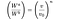Equation 13. Relationship between a gas’ microstate differences and volume differences for state a and b

Taken together, equations 12 and 13 may thus be interpreted as the entropy change for an ideal Boltzmann gas from state a (with v,T) to state b (with v₀,T) given by: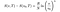Equation 14. The change in entropy for an ideal Boltzmann gas, expressed from Boltzmann’s relation (eq. 10)

Let ϕ(v,T)dv be the entropy density per unit volume in the frequency interval between v and v+dv. Then for energy density ρ (Pais, 1979):

Einstein begins by assuming that Wien’s law (eq. 9) is applicable. Then

If the radiation is contained in the volume v, then S(v, v, T) = ϕ×v dv and E(v, v, T) = ρ×v dv are the total entropy and energy in the volume in the interval [v, v+dv] , respectively (Pais, 1979). Then, assuming Wien’s law (eq. 5), one finds that the change in entropy is given by: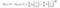Equation 17. Change in entropy expressed from Wien’s law (eq. 9)

Comparing the change in entropy expressed from Boltzmann’s relation (eq. 14) and the change in entropy expressed from Wien’s law (eq. 9), Einstein obtained his light-quantum hypothesis.

## Einstein’s Light-Quantum Hypothesis

Monochromatic radiation of low density behaves in thermodynamic respect as if it consists of mutually independent energy quanta of magnitude Rβv/N.

Einstein himself stated the above hypothesis as a theorem in his paper, even though it was based on Wien’s law which (although consistent with experiment up until that point) still needed to be proved from first principles (Pais, 1979). In Einstein’s own words (Einstein, 1905a pp. 143), translated: “If monochromatic radiation-of sufficiently low density behaves, as far as the volume-dependence of its entropy is concerned, as a discontinuous medium consisting of energy quanta of magnitude Rβv/N, it is plausible to investigate whether the laws on creation and transformation of light are also such as if light consisted of energy quanta.” (Ter Haar, 1967). In other words:

`"The light-quantum hypothesis is an assertion about a quantum property of free electromagnetic radiation; the heuristic principle is a tentative extension of these properties of light to the interaction between light and matter"- Excerpt, "Einstein and the quantum theory" by Abrahaim Pais (1979)`

On the basis of this ‘heuristic principle’, Einstein proposed the following simplest picture for the photoelectric effect:

A light-quantum gives all its energy to a single electron.

He also noted that an electron ejected from the interior of a black body will in general suffer an energy loss before it reaches the surface (Pais, 1979). Let Eₘₐₓ be the electron energy for the case where this energy loss is zero. Then, Einstein proposed the relation

Where h is Planck’s constant, v is the frequency of the radiation and P the work function, the energy needed to escape the surface of the body. Einstein notes that the equation (eq. 18) explains Lenard’s 1902 observation that electron energy does not show “the slightest dependence on light intensity”. The equation is also notable because it makes very strong predictions:

• First, it claims that the energy of individually ejected electrons increases linearly with the frequency of the light;
• Second, it claims that the slope of the (E,v) plot is a universal constant, independent of the nature of the irradiated material;
• Third, it claims that the value of the slope of the (E,v) plot is predicted to be Planck’s constant, determined from the radiation law.

In other words, Einstein in his paper theorized that the energy in each quantum of light was equal to the frequency of the light multiplied by a constant determined by the radiation law, now known as Planck’s constant h.

His prediction explained that the energy potential of a light source depends only on the frequency of light and not on its intensity:

• A low-intensity, high-frequency source of light can supply a few high energy photons which may generate the photoelectric effect; whereas
• A high-intensity, low-frequency source of light can supply no photons of sufficient individual energy to dislodge any electrons.

Einstein’s prediction was experimentally varified in 1914 by Robert Andrews Millikan.

## Aftermath

It was Einstein’s discovery of light particles, “photons”, and his predictions that photons above a certain threshold frequency are what ejects electrons in the photoelectric effect which arguably what led to the 20th century quantum revolution in physics. It also earned Einstein the 1921 Nobel Prize in Physics, which the Nobel committee stated should be awarded to Albert Einstein

“For his services to theoretical physics, and especially for his discovery of the law of the photoelectric effect.”